xWheel and axleEncyclopedia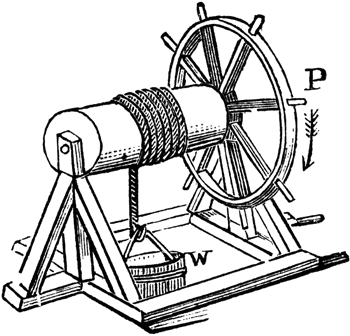The wheel and axle is one of six simple machine
Simple machine
A simple machine is a mechanical device that changes the direction or magnitude of a force.In general, they can be defined as the simplest mechanisms that use mechanical advantage to multiply force. A simple machine uses a single applied force to do work against a single load force...

s developed in ancient times and is in the category of a second- or third-class lever
Lever
In physics, a lever is a rigid object that is used with an appropriate fulcrum or pivot point to either multiply the mechanical force that can be applied to another object or resistance force , or multiply the distance and speed at which the opposite end of the rigid object travels.This leverage...

. In its simplest form it consists of a rod attached to a wheel so that their movements are coupled when one of the parts is turned. The wheel and axle is used either as a force multiplier (such as a doorknob, steering wheel or fishing reel) or as a distance multiplier (such as on a bicycle or the driven wheels of a car). In the first kind of application, the larger wheel is used to create more torque (in the axle) with less force. In the second kind of application, when the axle is turned, the outside of the wheel turns at a greater linear speed that is proportional to the ratio of the radii of the wheel and axle. For example, if a bike wheel has a gear that turns eight inches in one second, and the wheel circumference is eighty inches, the wheel rotates through a distance ten times greater than the gear (and reducing the number of rotations of the pedals required). By varying the radii of the axle and/or wheel, any amount of mechanical advantage may be gained.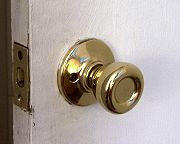## Description

The wheel and axle is a simple machine that is generally is classified as a lever and provides mechanical advantage. The mechanical advantage is the ratio of the resistance to the effort. It consists of a rod attached to a wheel so that their movements are coupled when one of the parts is turned. When the axle is turned, the outside of the wheel turns at a greater linear speed because the rotational speed is the same. This principle is used in cars to gain more distance by applying a large torque (from the engine) to the axle, causing the wheels, which have a much larger radius, to turn. In the reverse case, when a force is applied to the wheel, more torque is created with less force. The result is proportional to the ratio of the radii. For example, if a sailor is pushing a capstan
Capstan
Capstan may refer to:*Capstan , a rotating machine used to control or apply force to another element*Capstan , rotating spindles used to move recording tape through the mechanism of a tape recorder...

bar, pushing closer to the center is harder because he makes use of the wheel and axle as if it were a lever. Because the longer a lever is, the less force you have to use, the longer the bar the less effort is required.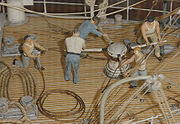## History

It is not certain who created wheel and axle, it is known that it was used in ancient times. The oldest wheel publicized by archaeologists was found in 2002 in Ljubljana
Ljubljana
Ljubljana is the capital of Slovenia and its largest city. It is the centre of the City Municipality of Ljubljana. It is located in the centre of the country in the Ljubljana Basin, and is a mid-sized city of some 270,000 inhabitants...

. Austrian experts established that the wheel is between 5,100 and 5,350 years old and is therefore at least a century older than those found in Switzerland and southern Germany. The wheel was made of ash and oak and had a radius of 70 cm. The axle is 120 cm long and made of oak.

## Misconceptions

One misconception about the wheel and axle is that any wheel on a cylinder is a wheel and axle. This is not so. To be a true wheel and axle, the wheel must be firmly attached to the axle so that if one is turned the other turns with it.

## Calculating mechanical advantage

The mechanical advantage of a simple machine like the wheel and axle is computed as the ratio of the resistance to the effort. The larger the ratio the greater the multiplication of force (torque) created or distance achieved. By varying the radii of the axle and/or wheel, any amount of mechanical advantage may be gained. In this manner, the size of the wheel may be increased to an inconvenient extent. In this case a system or combination of wheels (often toothed, that is, gear
Gear
A gear is a rotating machine part having cut teeth, or cogs, which mesh with another toothed part in order to transmit torque. Two or more gears working in tandem are called a transmission and can produce a mechanical advantage through a gear ratio and thus may be considered a simple machine....

s) are used. As a wheel and axle is a type of lever, a system of wheels and axles is like a compound lever.

### Ideal mechanical advantage

The ideal mechanical advantage of a wheel and axle is calculated with the following formula: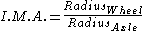### Actual mechanical advantage

The actual mechanical advantage of a wheel and axle is calculated with the following formula: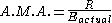where
R = resistance force
Resistance force
In physics, resistance force is the force which an effort force must overcome in order to do work on an object via a simple machine. Resistance force, like most other forces, is measured in newtons or in pounds-force....

, i.e. the weight of the bucket in this example.
Eactual = actual effort force, the force required to turn the wheel.

## Additional Resources

Basic Machines and How They Work, United States. Bureau of Naval Personnel, Courier Dover Publications 1965, pp. 3–1 and following preview online
The source of this article is wikipedia, the free encyclopedia.  The text of this article is licensed under the GFDL.